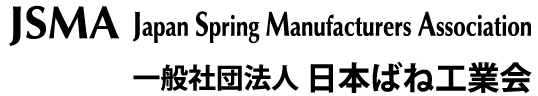New Alloy: Inconel® 617 is now available from Alloy Wire. Learn about Inconel® 617

# Designing a Tape Element

The method is similar to that used in designing a round wire element.

Where:

Example:

1.  To calculate the tape size and length required for a 600w/220v mica-wound element in a band heater, operating at a maximum temperature of 800°C, the total resistance of the element at operating temperature (Rt) will be:

2.  Using RW80 tape, the Temperature Resistance Factor (F) at 800°C is 1.051.  Thus, the total resistance of the element at 20°C (R) will be:

3.  Knowing the dimensions of the mica-board, the length of the tape that may be wound round it may be estimated.  Thus, the resistance required per metre of tape will be:

For example a length of tape of 7 metres –

4.  A tape size of 1.1mm x 0.09mm has a resistance per metre of 11.10 ohms/m (see stock sizes) which is near to 10.97 ohms/m.

5.  To verify the actual tape length (L)

A change in tape length may mean adding or subtracting turns (altering the pitch of the tape) to achieve the total resistance value required.

6.  To verify the surface area loading (S):

If your calculated surface area loading is too high or low (see table) you should re-calculate changing one or more of the following:

– The tape length and size